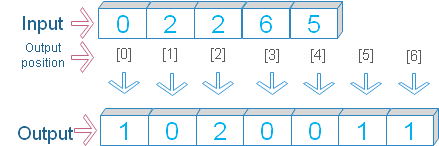# Numpy bincount()

Numpy

``numpy.bincount(x, weights=None, minlength=0)``
Return int ndarray .
 `x` Input array of non negative of 1 D `weight` (optional ), array of same size of input array. Weight of the elements to be used `minlength` Int (optional ), minimum length of bins

## Size of the return array

The length of output array is maximum value of input array + 1.If highest value of input array is 5 then there will be 6 elements in output array.

The output array will show number of occurrence of elements starting from 0 to highest value of the input array.

## Examples 1``````import numpy as np
ma=np.array([0,2,2,6,5])
print(np.bincount(ma))``````
Output
``[1 0 2 0 0 1 1]``
If the input array has the highest value of 6, then our output array will have 7 elements. Each element of our output array will show frequency of occurrence of numbers starting from 0 to highest number.

## Example 2

Using weight
``````import numpy as np
my_array1 = np.array([0,2,2,6,5])
my_array2 = np.array([2,2,1,3,4])
my_bin=np.bincount(my_array2,weights=my_array1)
print(my_bin)``````
Output
``[0. 2. 2. 6. 5.]``

## Example 3

``````import numpy as np
my_array1 = np.array([0,2,2,6,5])
my_array2 = np.array([2,2,1,3,4])
my_bin=np.bincount(my_array1,my_array2)
print(my_bin)``````
Output
``[2. 0. 3. 0. 0. 4. 3.]``

## Example 4

``````import numpy as np
my_array1 = np.array([0,3,3,5])
my_array2 = np.array([2,1,4,6])
my_bin=np.bincount(my_array1,my_array2)
print(my_bin)``````
Output
``[2. 0. 0. 5. 0. 6.]``

Subscribe to our YouTube Channel here

## Subscribe

* indicates required
Subscribe to plus2netplus2net.com